College Physics : Radioactive Decay

Example Questions

Example Question #1 : Radioactive Decay

Radiocarbon dating is a method that allows scientists to estimate the age of organisms after they have died. A fairly constant amount of radioactive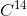remains in the animal while alive, but once it dies thedegrades over time into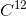. By measuring the relative amount of these two in a dead organism compared to a living one,  along with knowing that the half-life ofis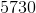years, it can be determined how long ago the organism died.

If a scientist finds that a fossil contains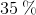amount of thenormally found in the atmosphere at that time, approximately how old is this fossil?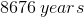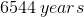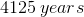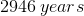Explanation:

In this question, we're given a brief description of radiocarbon dating. We're given the amount ofthat has been found in a fossil sample and we're asked to find the approximate age of the fossil.

First, let's briefly go over radiocarbon dating. This method essentially assumes that the amount of radioactive carbon within an organism remains fairly stable at any given time while the organism is alive. Moreover, this amount of radioactive carbon is related to the amount of radioactive carbon in the atmosphere. Once the organism dies, however, it ceases to gain any radioactive carbon; rather, thethat was present now begins to decay into. Thus, by measuring the amount ofin the organism and comparing it to the amount in the atmosphere, the age at which the organism died can be approximated.

Since we're dealing with the decay of, this is a radioactive decay problem. Recall that all radioactive decay reactions follow first-order rate kinetics. What this means is that the rate of decay is only dependent on the amount of radioactive material at any given instant. Hence, we can use the first-order rate equation.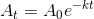We can further rearrange this expression to isolate the variable for time.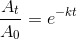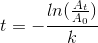In arriving at this expression, we see that we need to know the rate constant,, in order to solve for. To do this, we can use the equation that relates the half-life to the rate constant for a first-order process.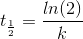Rearranging, we can find the rate-constant.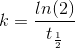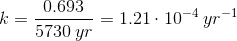Now that we have the rate constant, we can plug this value into the previous expression to solve for.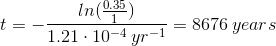Example Question #13 : College Physics

Suppose that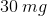of a radioactive drug is injected into a patient. Afterminutes pass by, another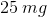is injected. After anotherminutes pass by, how much of the drug is expected to be in the patient's body?

Note: The half-life of the drug isminutes.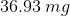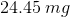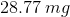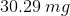Explanation:

For this problem, the first step is to find the rate constant for the decay reaction. Since we're given the half-life, we can calculate this value using the following equation.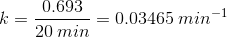Now, we can use the first order rate equation to find out how much drug will be left after the first time interval ofminutes.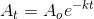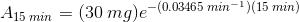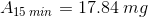So, after the firstminutes, there will be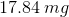of the drug in the patient's body. But from the question stem we're told that an additionalof the drug is injected. Hence, there is now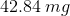of the drug present.

With this new amount in mind, we'll need to calculate how much of the drug will be present after more time has elapsed. We can do this by using the same equation as before.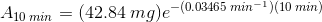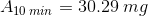This is the final amount of drug that is expected to be present in the patient's body at that instant of time.

Example Question #11 : College Physics

Iodine-131 has a half life of 8.02 days and undergoes beta decay.

If asample of iodine-131 is left out for 1 week (7 days), how much of the sample will still be iodine-131? What will Iodine - 131 decay into?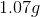of iodine-131 will be left and will decay into antimony-127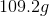of iodine-131 will be left and it will decay into xenon-131of iodine-131 will be left and it will decay into xenon-131of iodine-131 will be left and it will decay into antimony-127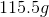of iodine-131 and decays into tellurium-131of iodine-131 will be left and it will decay into xenon-131

Explanation:

Finding the amount is a simple half life problem. Start with setting up what you know for any half-life problem.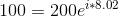,is the rate of decay, 200 is the initial amount, and 100 is the half left after 8.02 days.

From there simplify.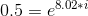. To solve this take the natural log of both sides so that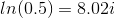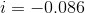Now plug this into the equation and find what happens after 7 days.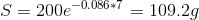Beta decay is when a neutron releases an electron and becomes a proton. Therefore, the atomic number goes up by one but the atomic mass remains the same. This results in xenon-131.

All College Physics Resources